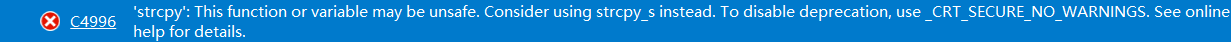2021-08-25 16:40

# C++使用strcpy函数时编译器报错

``````//这是报错代码
#include<bits/stdc++.h>
using namespace std;

int main()
{
char ch = "character string";
const char* ch1 = "character";
char* ps;
cout << ch << "and";
cout << ch1 << endl;
cout << "Enter:";
cin >> ch;
ps = ch;
cout << ps << "!\n";
cout << "Before using strcpy():\n";
cout << ch << "at" << (int*)ps << endl;
//开辟内存
ps = new char[strlen(ch) + 1];
strcpy(ps, ch);
cout << "After using strcpy():\n";
cout << ps << "at" << (int*)ps << endl;
//释放内存
delete [] ps;
return 0;
}

``````• 收藏